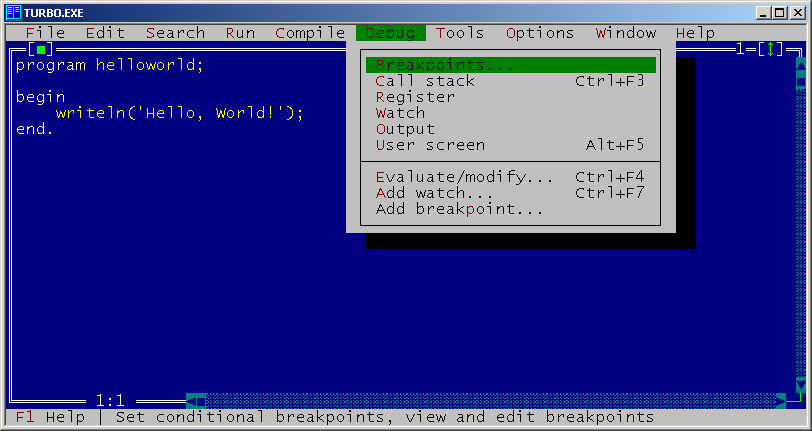# Turbo Pascal 7.0

Version of implementation Turbo Pascal of programming language Pascal

This is the last version of Turbo Pascal, released on March 9, 1993.

The compiler became cross-platform — it allowed compiling programs for DOS and for Windows. Another major feature is the possibility of creating DLLs.Turbo Pascal 7.0

## Examples:

### Factorial - Pascal (44):

This example uses recursive factorial definition.

Note that this example works in all given implementations of Pascal, but it produces slightly different results. In gpc everything works perfectly. Turbo Pascal and Free Pascal have arithmetic overflow for factorial of numbers greater than 12, but Free Pascal reports an error:

13! = Runtime error 215 at \$004013C7
\$004013C7
\$00401449
\$004063E0

while Turbo Pascal doesn’t detect the error and simply prints wrong values:

13! = 1932053504
14! = 1278945280
15! = 2004310016
16! = 2004189184

This example doesn’t work in Turbo Pascal 3.0 and earlier due to absence of `longint` data type in these versions.

In GNU Pascal this program works without any problems.

``````program factorial;

function fact(n: integer): longint;
begin
if (n = 0) then
fact := 1
else
fact := n * fact(n - 1);
end;

var
n: integer;

begin
for n := 0 to 16 do
writeln(n, '! = ', fact(n));
end.
``````

### Fibonacci numbers - Pascal (58):

This example uses recursive definition of Fibonacci numbers.

``````program fibonacci;

function fib(n:integer): integer;
begin
if (n <= 2) then
fib := 1
else
fib := fib(n-1) + fib(n-2);
end;

var
i:integer;

begin
for i := 1 to 16 do
write(fib(i), ', ');
writeln('...');
end.
``````

### Factorial - Pascal (96):

This example is exactly the same as main recursive example for Pascal implementations, except for that it uses `real` data type to store factorial values. Command `writeln(f:-1:0)` outputs the floating point number `f` with 0 digits after decimal point and left-justifies it.

``````program factorial;

function fact(n: integer): real;
begin
if (n = 0) then
fact := 1
else
fact := n * fact(n - 1);
end;

var
n: integer;

begin
for n := 0 to 16 do
writeln(n, '! = ', fact(n):-1:0);
end.
``````

### Hello, World! - Pascal (57):

``````program helloworld;

begin
writeln('Hello, World!');
end.
``````